# Quarter Circles: Calculating Area, Perimeter & Radius

Lesson Transcript
Instructor
Yuanxin (Amy) Yang Alcocer

Amy has a master's degree in secondary education and has been teaching math for over 9 years. Amy has worked with students at all levels from those with special needs to those that are gifted.

Expert Contributor
Robert Ferdinand

Robert Ferdinand has taught university-level mathematics, statistics and computer science from freshmen to senior level. Robert has a PhD in Applied Mathematics.

A quarter-circle is formed by taking one part of a circle which has been equally divided into four. Learn about the quarter circle and understand how to calculate its area, perimeter, and radius. Updated: 11/08/2021

## A Quarter Circle

Meet the quarter circle.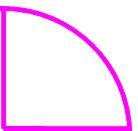It is one fourth of a circle. If you take a whole circle and slice it into four pieces, then one of those slices makes a quarter circle.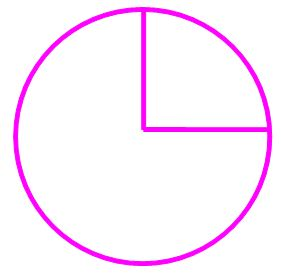In this lesson, we will look at finding the area, the perimeter, and the radius of a quarter circle. Knowing how to find these key pieces of information for quarter circles will serve you well as you advance in your math classes and as you take standardized math tests. Not only will you find these types of problems on math tests, but you may also find these types of problems working as an architect or as an engineer.

So let's get started.An error occurred trying to load this video.

Try refreshing the page, or contact customer support.

Coming up next: Circles in Word Problems

### You're on a roll. Keep up the good work!

Replay
Your next lesson will play in 10 seconds
• 0:00 A Quarter Circle
• 0:35 Calculating Area
• 2:15 Calculating Perimeter
• 6:05 Lesson Summary
Save Save

Want to watch this again later?

Timeline
Autoplay
Autoplay
Speed Speed

## Calculating Area

First, let's talk about area. Normally, with these types of problems, you will be given the radius of the circle. Look carefully at your quarter circle and you will see that the two straight sides are both a radius of the circle. So, you only need the measurement of one of these straight sides. The other side will be the same.

Let's work through a problem together. Let's say the problem tells you that one of the straight sides of the quarter circle measures 3 inches, and it wants you to find the area of the quarter circle. How would you go about finding the answer?

Well, you think about what the quarter circle is. It is one fourth of a whole circle. So, you can find the area of the whole circle and then divide by 4 to find the area of your quarter circle. You know that the area of a whole circle is found by using the formula A = pi * r^2, where you square your radius and multiply it by pi. You can do the same for this problem and then divide this number by 4 to find your answer.

Using the formula and plugging in 3 for r, you get A = pi * 3^2 = 9 * pi = 28.26 inches squared. So the area of the whole circle is 28.26 inches squared. Now, to find the area of the quarter circle, you divide the 28.26 by 4 to get 28.26 / 4 = 7.065 inches squared. So your answer is 7.065 inches squared.

Remember that your area units are always squared. That is why your answer includes the inches squared at the end.

## Calculating Perimeter

Now, let's calculate the perimeter. We will work off the same problem where the radius is 3 inches. You might think that all you have to do is to divide the perimeter or circumference of the whole circle by 4 to find your answer, kind of like what you did for the area. You are close, but you are missing part of your answer.

Look carefully at your quarter circle: what do you see in addition to a quarter of the circumference of the whole circle? Remember the circumference of the whole circles gives you that outer ring. What else do you have in your quarter circle that you don't have in your whole circle? That's right, you have the two straight sides.

So, to find your circumference, you can find a quarter of the circumference of the whole circle first. This will give you the length of the curve. Then you can add the two straight sides to find your answer. Remember, your two straight sides are both the radii of your circle, so their lengths will be the same as the length of the radius that is given.

For our problem, you remember that the formula for finding the perimeter or circumference of a whole circle is C = 2 * pi * r. You multiply the radius by 2 and pi. For our answer, we will again leave it in simplified fraction form and with the pi. So, your radius measures 3, so the circumference of the whole circle is C = 2 * pi * 3 = 6 * pi = 18.84 inches. Dividing this by 4 gives you 18.84 / 4 inches = 4.71 inches. This is only the curved part, so now you add the straight sides to this measurement.

You have two straight sides that both measure 3, so you add 4.71 + 3 + 3 = 10.71 inches. So, your perimeter of your quarter circle is 10.71 inches.

To unlock this lesson you must be a Study.com Member.

## Practice Questions

1. A quarter circle has area = 26π square centimeters (cm^2). Find the radius of this quarter circle.

2. Find the whole perimeter of a quarter circle whose radius is 5 inches.

1. The area A of a quarter circle of radius r is one-fourth of the area of the whole circle of radius r. Hence the area of the quarter circle will be given by the following formula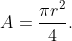The question gives us A = 26π and asks us to find r. Using the formula above, we get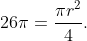Multiplying both sides of the above equation by 4 and then dividing both sides by π yields the radius of the quarter circle as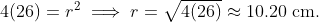2. The perimeter P of a whole quarter circle equals the perimeter of the curved part of the circle, which is one fourth the perimeter of the whole circle, added to 2 times the radius r of the circle.

Hence,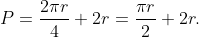Since r = 5 inches from the question, we substitute in the above formula to get the whole perimeter of the quarter circle as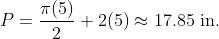### Register to view this lesson

Are you a student or a teacher?